﻿ Inter-Relation between Laser Induced Photoconductance and Millimeter Wave Absorption Using C-Si Intrinsic Resistivity

# Inter-Relation between Laser Induced Photoconductance and Millimeter Wave Absorption Using C-Si Intrinsic Resistivity

Roy B*, Tsui A, Oni O and Vlahovic B
Department of Mathematics and Physics, North Carolina Central University, Durham, North Carolina, USA
*Corresponding Author: Roy B, Department of Mathematics and Physics, North Carolina Central University, Durham, North Carolina, 27707, USA, Tel: 919-599- 2043, Email: [email protected]

Received Date: Jan 06, 2018 / Accepted Date: Jan 24, 2018 / Published Date: Jan 31, 2018

### Abstract

In a contactless photo conductance measurement system the radio-frequency (RF) probe transmission (ΔV/ V0) should be proportional to the product of laser-induced carrier concentration and carrier mobility (ÃÂΣμ) through a sensitivity factor (A). We use 532 nm laser (pump)-millimeter wave (mmw-probe) system whose concentrations (ÃÂ) are calculated by considering single-surface reflection of the laser beam and mobility (Σμ) derived from a model. In order to ascertain A we use five c-Si (100) samples having resistivity in the range 15-130 Ω-cm. For relating (ΔV/V0) with ÃÂΣμ to find A, we take their ratio and quantify A once using a quadratic-fit functional form of the ratio of sample resistivity to air resistivity (ρ/ρ0), and another time using product of free-space impedance and sample thickness (ρ/Z0t). A is ascertained for (ΔV/V0)-laser fluence linear region while fluence is in range 0-1.7 μJ/cm2 and probe frequency is fixed at 140 GHz. Value of A is further fine-tuned by multiplying with 0.85 (to linearize the ratio with the non-dimensional function) and finally obtain sensitivity A=0.291. Standard error in mmw photo conductance (obtained using calculated A) between the two approaches diminish with laser attenuation roughly at a rate ± 0.53 × 10-5 S, per decimal neutral density filter size.

#### Introduction

Accuracy of laser pulse induced carrier density estimate is an important parameter for studying photoconductance quantitatively in photo condensed media . Its decay process is well studied using noncontact methods such as laser pump, microwave/THz probing. In order to relate the product of excess minority charge carrier concentrations and mobility (ϕΣμ) in materials at a given pump fluence, there is a need to have very accurate estimate of sensitivity factor that depend upon the relationship between the actual probe power transmitted through sample converted to a detected voltage. Transport mechanism in dielectrics and semiconductor targets under ultra-short pulsed laser irradiations are complicated, and most of the users rely on modeling . It is also reported that for thin silicon films the reflectivity (R) strongly depends on carrier density and pulse duration. Visible laser as a pump, and microwave or THz beam as probe have been widely used for different photovoltaic materials and probe responsivities have been used to calculate photovoltaic charge dynamical estimates. This has advantage to solar performance evaluation of the material in non-contact procedures. There is dearth of published data on photoconductance based on estimates of charge mobilities-carrier density product measured in non-contact fashion using mmw transmission when probing wavelengths are in the range 1.8 mm-2.7 mm. Experimental studies to infer free carrier absorption (FCA), two-photon absorption (TPA) and thermally induced absorption enhancement (TAE) have proved that FCA is the most dominant cause of non-linearities in probe signal transmission (T) . Silicon sample uniformity also bears a strong relationship with the time-resolved signal, and it is reported that light pulse at 532 nm yields non-uniform distribution of excess carriers . Distribution of excess carriers is well described by Beer’s law for two parallel plates neglecting internal interference but considering multiple reflections [4-6].

Temporal decay of the conductance signal through the material represents the lifetime of the photo-generated carriers. Time dependence of absorption of mmw power (P) and corresponding timedependent photoconductance in sample are related by the sensitivity (A) in the probe transmission to ϕΣμ relation [5-7]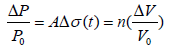(1)

where ΔP is the millimeter wave power absorbed and P0 is the initial mmw power appearing on the detector horn antenna when probe beam is passing through sample, but laser is turned off (no photoconductance in sample). Conductivity σ=qed ϕΣμ, where qe is electron charge and d is laser penetration depth, A is the sensitivity factor, and n is the exponent in the probe beam power converted to voltage by the detector, P=Vn. The constant A in eqn. (1) primarily depends upon this probe power-voltage conversion factor ‘n’, and might also incorporate apparatus geometry, material-medium interface and sample properties .

Martin et al.  points out using microwave reflection technique that effective lifetime calculations in Si is better obtained under low injection conditions. Swiatkowski et al.  incorporated a timedependent decay behavior into the right-hand side (RHS) of eqn. (1) to account for decay of principal mode distribution in Si wafers due to existence of lateral non-uniformity in photoconductivity that arise due to non-uniformity in initial induced carrier distribution. Lui and Hegmann  have formulated a simple time-dependent carrier density model incorporating use of normalized change in THz probe power and show that the free carrier density is a function of pumpprobe delay time. Crothers et al.  have investigated the dependence of charge carrier mobility and recombination mechanisms that result from intrinsic properties of perovskite. In that investigation they have used pump R and T and used radiation model approach  to compute the photo-excited carriers (Nc). Our approach utilizes a similar method, but incorporates use of measured pump fluence obtained at various pump intensities that bear a linear relationship between mmw transmissions, assuming all photons those are not reflected by the first surface are completely absorbed by sample resulting in free carriers.

For the first time, a sub-mm (D band: 110-170 GHz) coherent source (0.45 to 0.70 meV range) probing apparatus with a 532 nm, 0.69 ns pulse-width pump laser operated with a repetition rate of 1 KHz is developed in house. This allows to successfully store voltage transient information from inorganic, hybrid, and organic photovoltaic materials at a very high sampling rate in the transmission mode i.e., probe beam completely passing through sample. In this work, steadystate, and transient data are collected with the 140 GHz probe beam to estimate the sensitivity A. Non-dimensional quadratic functional form of intrinsic resistivity of the 5 Si samples are used to derive A, and then adjust till a slope is unity after taking ratio of mmw voltage transmission and the carrier-mobility product with constants applied. Silicon is selected because its properties are well known and it has quite reliable mobility models.

In the following sections, we describe experimental approach, methodology to estimate charge carrier density estimates and comparisons with Crothers et al.  radiation method. A short discussion is included about the discrepancy, and possible sources of error. This is followed by presenting results of correlating various experimental and derived parameters used in the transmission mode, description of non-dimensional form of resistivity and sample resistances, estimation procedure for sensitivity constant A, and photoconductance variation with corresponding laser power.

#### Experiment And Analysis

Photo-induced charge carrier concentration

Czochralski grown crystalline P-doped silicon (c-Si) has an energy gap of 1.11 eV at room temperature hence we use 532 nm (2.3 eV) pulsed laser energy to create carrier concentration enough to cause changes in the electric field that cause probe signal absorption and record the time response of the absorbed power signal over the pumpprobe delay.

These wafers have resistivity in the range 15-130 Ω-cm. Pulsed laser fluence at 532 nm with a beam cross section of 78.54 mm2 made to be incident on sample at an incidence angle of 65.40. This creates an elliptical laser (pump) spot of size 86.39 mm2 on sample plane. Before selecting the 5 c-Si samples (Si-2,4,7,8, and 10) out of 11 different wafers, we consider the probe beam transmission criteria (>60%) using the dc response of the beam from a Schottky zero-bias detector diode (ZBD). Si-2 is the only N type semiconductor, and the rest are Boron doped (P) type. Table 1 gives the details about the 5 c-Si samples we have used to obtain the charge carrier concentration and mobility for use in sensitivity analyses.

Sample Wafer Thickness (µm) Transmission at 140 GHz (%) Type Resistivity (Ω-cm)
Si-2 525 66 N 50.05
Si-4 512-538 64 P 16.88
Si-7 500 67 P 23.69
Si-8 725 61 P 127.56
Si-10 529 77 P 40.74

Table 1: c-Si details with probe beam transmission (%) taken using the dc response of sample using zero-bias.

Schottky detector after passing V-polarized Cavity stabilized IMPATT (Impact Ionization Avalanche Transit Time) Diode Oscillator (CIDO) beam through sample. Resistivity for each sample obtained using 4-point probe technique.

Net charge carrier density estimates are based on the laser beam energy computed photon flux, and by applying the penetration depth d=1/α, alpha being the absorption coefficient of c-silicon (7850 cm-1) at the pump wavelength 532 nm [10-13].

Transmitted laser power (Tpump) used for Crothers et al.  second surface reflection related transmission is calculated using measured reflection (Rpump) and using the film thickness of 1 μm through which laser beam penetrate.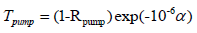(2)

Rpump is a measured quantity obtained using a well calibrated laser power meter. We compute the beam energy by using the Rpump (considering minimum absorption), the measured beam power (Pnd) for different neutral density (ND) filter settings 0.9 to 2.2 using the same power meter. A beam energy of 8.71 μJ (at 1 KHz repetition rate) corresponds to laser fluence 10.08 μJ cm-2 and excess carriers so created due to laser fluence over Si surface undergo diffusion and drift processes to the extent of laser penetration depth (1 μm). Hence, we compute the sheet carrier concentration, by assuming that 100% of photons are converted to minority carrier numbers. Using beam energy, the photon count is computed using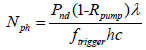(3)

Pnd is the laser power measured exactly at sample location when neutral density filter set between 0.8 and 2.2. λ is laser wavelength, trigger frequency (ftrigger) is fixed at 1 KHz, ‘h’ is Planck’s constant and ‘c’ is velocity of light in vacuum Figure 1 below shows the comparison of photoexcited charge carrier (Nc=Nph) computed once multiplying the right side of eqn. (3) by the (1-Tpump) terms  and once neglecting it. Histogram of the photon-count difference data shows that there is a peak at around 0.2 × 1012 and this spans a range of laser fluence in range 0 to 1.7 μJ/cm2 (ΔV/V0 – fluence linear region). Over the entire laser power range, the carrier concentrations are 22% lower using (1-Tpump) factor in eqn. (3) than not using the term and considering a single pass thus neglecting absorption in only the top 1.274 μm layer. Eqn. (3) is justified by assuming that there is no transmission of laser through sample and all transmitted photons (1-Rpump) give rise to Nph (efficiency 100%) resulting in equal number of charge carrier (electron, and hole) Nc.

Figure 1: Photon count in beam Nc on c-Si samples for entire range of laser fluence 0-10 μJ/cm2 once considering Nc= N ph (1-Tpump) shown in x-axis and another time only Nph. Inset: histogram of the difference in carrier population in these two approaches. Dotted line in plot is the fit of comparison and solid line is represent 1:1.

Millimeter wave probe and laser data

For moderately low intrinsic conductivity material, we estimate mmw probe power absorbed following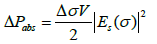(4)

where V is the sample volume and Es(σ) is electric field through sample as function of conductivity. The factor 1/2 comes from time average of the square of a sinusoidal function. If the absorption of the probing field in the sample is small, then the field in the sample is approximately the same as the transmitted field except for the effect of the refractive index change in the material neglecting reflection loss when the probe beam exits the sample. Using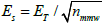and substituting for ES in eqn. (4) yields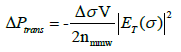(5)

Subscript ‘trans’ denotes transmitted probe power through sample, and subscript ‘nmmw’ is the index of refraction of c-Si samples which is 3.41 for the 110-170 GHz probe frequency. Volume, V=ad, where a is sample area and d is sample thickness. In our case it is the penetration depth (d) of the laser beam which is depth from surface where intensity falls off by a factor of e, and it depends upon the absorption coefficient of the material for the given energy of photon in electron-volts) and ‘a’ the sample area. Laser pulse photons that exceed the bandgap energy of the material are absorbed and are responsible for the excess number of electron-hole pairs in sample. Multiplying and dividing by ε0c (permittivity of free-space times velocity of light), we obtain the expression in brackets below which is simply the transmitted power, Ptrans, as computed from the Poynting vector.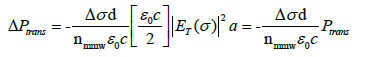(6)

We rewrite eqn. (6) as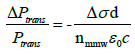(7)

Δσd can be written as change in conductance ΔG, introducing again the mmw power to voltage conversion factor ‘n’ [5-7] in eqn. (7) power transmission can be equated to voltage transmission (ΔV=RF change in voltage when laser is on, V0 is direct current response through sample when laser is turned off) and related with constant (C) times ΔG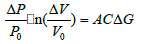(8)

Essentially A=(1/n) c.f. from eqns. (1) and (8), C being the constant 1/ (nmmwɛoc), and n is the millimeter wave power to voltage transformation factor (typically found between 1 and 2 for microwaves ). In eqn. (8) we can re-write the change in conductance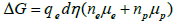, where ne, and np are laser-induced concentrations (cm-3) and μe and μp being the carrier mobility (cm2/V-s) for electron and hole respectively,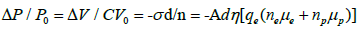(9)

Quantum efficiency of photon to carrier conversion (η ) is considered to be 1 in our analysis. We will represent LHS and RHS of eqn. (9) as ΔV/(V0Cqed) and (neμe+npμp)=ϕΣμ, respectively. Ideally, taking the ratio LHS/RHS should yield a proportionality constant A (≈1/n) same for all samples. The RHS is also represented as the product of respective laser power derived carrier densities and (cm-3) and PV lighthouse calculator [14,15] mobility expressed in cm2/V-s and denoted as ϕΣμ here.

To estimate A, we use millimeter-wave (mmw) technique for measuring photo conductance and decay in these samples. Schematic of this arrangement is shown in Figure 2. A fixed (140 GHz) cavity- IMPATT diode oscillator (CIDO) used as probe source with quasioptical arrangement, a horn antenna fed Schottky zero bias detector, a low noise amplifier and digitizer to store the single-cycle, or averaged transient signals. Photo-induced free carriers in c-Si sample vibrate according to the E field of the mmw probe signal which induces polarization, thereby causing a change in effective dielectric constant resulting mmw power absorption. The power absorption profile is recorded as voltage change in Schottky detector diode RF output which is amplified, digitized and averaged.

Figure 2: Quasi-optical measurement schematic (not to scale) of the CIDO mmw probe technique in which 5 crystalline silicon (c-Si) samples are used to collect experimental data. Transmission of mmw through top 1 μm sample. Schematic shows use of quasi-optical elements H- mmw horn antenna, C- TPX collimator lens, P-polarizer (vertical E field allowed to pass to match vertical polarization of 2.33 eV laser), S- Mylar beam-splitter, F- TPX focus lens, ZBD-zero bias Schottky diode detector (0-6GHz with responsivity 3.5V/mW) having two separate outputs for RF and dc voltages, LNA-low noise amplifier, DMM-digital multimeter for measuring dc voltages.

aLaser intensity I=I0/10ND.

We consider measured millimeter wave transmission ratio ΔV/V0, charge carrier densities (ne and np, cm-3) equated to Nc and assuming equal number of ne and np generated for each laser shot. We then use PV lighthouse calculator derived electron- and hole mobility (cm2/V-s). The calculated laser derived ne, and np and model derived mobility are then used for this analysis. These are given in Table 2.

Sample Resistivity
(Ω-cm)
NDa , Photon density
(Nph=ne =np ) (cm-3)
PVL mobility electron µe
(cm2/V-s)
PVL mobility holes µh
(cm2/V-s)
Probe -|ΔV/V0|
Si-2 50.05 0.9, 2.0722 × 1016 1064 410.2 0.0164
1.3, 8.9427 × 1015 1181 431.5 0.028
1.6, 4.055 × 1015 1263 445.6 0.0133
1.9, 2.2259 × 1015 1308 453 0.0125
Si-4 16.88 1.3, 9.4169 × 1015 1160 428.9 0.0118
1.6, 4.6598 × 1015 1229 441.6 0.0208
1.9, 2.8789 × 1015 1264 447.9 0.0064
2.2, 1.491 × 1015 1287 454 0.0136
Si-7 23.69 0.8, 2.4061 × 1016 1036 405 0.0137
0.9, 2.0914 × 1016 1057 409.2 0.0071
1.0, 1.627 × 1016 1094 416.2 0.0126
1.3, 9.2979 × 1015 1166 429.7 0.0036
1.6, 4.4786 × 1015 1239 442.9 0.0069
1.9, 2.6744 × 1015 1276 449.5 0.0068
Si-8 127.56 0.9, 2.0857 × 1016 1063 410.1 0.0035
1.2, 1.0387 × 1016 1161 428.3 0.0062
1.5, 5.8216 × 1015 1227 440 0.0017
1.8, 2.8602 × 1015 1288 450.4 0.0038
2.1, 9.7357 × 1014 1346 460.1 0.0039
Si-10 40.74 1.0, 1.5737 × 1016 1102 417.6 0.0022
1.3, 8.8955 × 1015 1176 431.2 0.000958
1.6, 4.1666 × 1015 1252 444.7 0.0013

Table 2: Sample-wise data for each of the ΔV/V0-fluence linear operating points where PVL resource is used to compute mobility.

Parameter correlation table and sample resistivity

Probe signal based mmw-transmission (LHS) is a measured quantity, and RHS of eqn. (9) is semi-empirically determined. We justify the present attempt to inter-relate them by first studying a correlation table for all the data pairs with each other. Non-dimensional quadratic form of sample intrinsic resistivity is used for the inter-relation. Table 3 below gives the Pearson’s correlation coefficients for sample size N=22 considering magnitudes only. The strong correlation coefficients are shown with bold numbers.

V0(volt) ΔV
(volt)
φΣμ (mVs-1) LHS/RHS ND Ρ (Ω-m) T (m) µ (m2/Vs) Ne
(m-3)
Fluence (µJ/cm2)
ΔV/V0 0.267 0.973 0.826 0.351 -0.826 -0.211 -0.293 -0.832 0.718 0.835
V0 -0.093 0.033 0.662 -0.030 0.939 -0.926 0.046 -0.406 0.0007
ΔV 0.898 0.207 -0.887 -0.055 -0.139 -0.891 0.665 0.896
φΣμ -0.133 -0.951 -0.057 -0.112 -0.984 0.537 0.988
LHS/RHS 0.040 -0.401 -0.467 0.103 0.335 -0.084
ND 0.043 0.112 0.969 -0.575 -0.944
ρ 0.971 0.135 -0.381 -0.078
t 0.176 -0.448 -0.116
µ -0.588 -0.976
Ne 0.533

Table 3: Gives the Pearson’s correlation coefficients (r) between measured, and modeled silicon transport parameters (N=22) used in the model defined in Equation 9 and subsequent attempt to interrelate the LHS and RHS of Equation 9 using quadratic functions of non-dimensional intrinsic resistivity at a fi × ed probe CIDO frequency of 140 GHz after varying pump(laser) powers. Strong r values are shown in bold numbers. All parameters are correlated after taking the magnitude of the observations or model outputs.

Millimeter wave transmission is highly sensitive to the RF change in power (voltage) at various laser power (r=0.97), correlations between measured mmw probe transmission (ΔV/V0) and carrier-mobility product, and laser fluence is also moderately strong, but they both bear strong negative correlation with laser ND and carrier mobility. We also note a strong correlation between the DC voltage |V0| with resistivity, and strong negative correlation with sample thickness. Usually, there is a negative sign associated with more DC power into the detector but, actually all ‘r’ data presented here are based on correlation between absolute values. Product of laser energy derived carrier concentration with PVL modeled mobility (ϕΣμ) does not show a good correlation with LHS/RHS however, it bears strong correlation with laser fluence and PVL mobility alone.

The results mentioned here are used to justify and establish an inter-relation between the probe signal transmissions with the semiempirically derived carrier-mobility product. ϕΣμ being the only variable part of RHS of eqn. (9). Strong positive correlated resistivity and negative correlated sample thickness are used in non-dimensional form explained below.

In order to compute the non-dimensional resistivity, sample resistivity for each wafer (ρ) (mentioned in Table 1) is normalized by air resistivity (ρair) 2 × 1016 Ω-m and then quadratic fit is obtained by fitting with the ratio LHS/RHS for each instance. Figure 3a shows the quadratic fit obtained using the coefficients. Figure 3b shows the similar quadratic fit but using the non-dimensional quantity ρ/(tZ0), where ‘t’ is the thickness of sample (m) and Z0 is air impedance (377 Ω).

Figure 3a and 3b: 3a shows the quadratic fit for LHS/RHS versus non-dimensional resistivity using ρair, with norm of residuals=0.327, and 3b shows the same using product of wafer thickness and air impedance Z0=377 Ω, with norm of residuals=0.355. Note: Irrespective of the non-dimensional group being used, the points on both the points on plot are almost identically located in terms of the ordinate (LHS/RHS).

The coefficients of the quadratic fit between the non-dimensional quantities (ρndsampleair, Ωndsample/(tZ0)) shown in Figures 3a and 3b are then used to estimate functions which we denote as Q1 and Q2 to operate on the product ϕΣμ to equate to the LHS.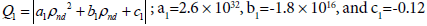(10)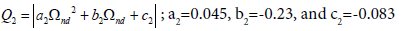(11)

Using these non-dimensional quadratic fit and employing the evaluated coefficients, and considering Eqn 1 we can equate the following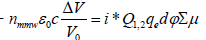(12)

Magnitudes of Q1,2 found to have a very weak oscillation with laser power computed for each of the silicon data and it is found to vary in the range -0.05 to -0.1. Combining eqns. (8) and (12) we can say that A should resemble the ratio A=i|Q1,2 |=1/n. After plugging in the numerical values of Q1,2 the LHS/RHS slope still need to be linearized hence, adjusted iteratively to find ‘i’. The constant multiplier ‘i’ was estimated to be 0.85. A is preliminarily evaluated as -0.291. In the next section, we will note the dependence of the error between sample photo-conductance derived by applying A=0.291.

Photoconductance (ΔG) using calculated sensitivity

Using right hand side of eqn. (12) we compute change in photoconductance (ΔG) by considering the evaluated sensitivity iQ1,2=- 0.291 as given in previous section, we attempt to plot the differences between ΔG computed using non-dimensional resistivity factor Q1 and another time using non-dimensional form of resistance Q2, as a function of laser power attenuation filter size (neutral density filter). The plot of the differences in Siemens (S) as function of attenuated incident laser power are shown in Figure 4a below, and subsequently, the LHS/RHS ratio are plotted as function of laser power in Watts and spline-interpolated (Figure 4b).

Figures 4a and 4b: 3a Shows the photoconductance (in Siemens) change data computed using Equation 12 after iterating i for slope=1. The data reveals reduced standard error (std. dev./√N)in ΔG data with decrease in laser power. Out of 11 laser attenuation levels (ND) shown here no. of samples N are 3, 2, 4, 4 and 3 corresponding to ND equal 0.9, 1.0, 1.3, 1.6 and 1.9 respectively, for other attenuation levels N=1; 3b shows the spline-interpolation plot of the LHS/RHS data as function of laser power (=I0/10ND). Note: magnitude in axes of 4a and 4b has an opposite sense, 4a has ND size and 4b has laser power directly assigned.

The photoconductance using (ΔG as in eqn. (8)) correlate strongly with the mmw transmission (r=0.905 for Q1; r=0.920 while using Q2); The mean of ratio (Q1/Q2) for all 22 laser attenuation levels using eqn. (10) and (11) for different sample resistivity yield 0.98, thereby signifying that there is a 2% difference in magnitudes between the use of Q1 and Q2 non-dimensional function. Hence, the error we see in Figure 4a that diminish with laser attenuation may be due to a) error in sample thickness and carrier concentration from laser fluence estimates, b) uncertainty in laser reflection (%) from sample surface, c) millimeter wave control volume being 97% of the actual due to laser being incident on sample at incidence angle 650.

The standard error between the ΔG estimates using the 2 methods (Eqns. 10 and 11) diminishes with laser power (Figure 4a) roughly in the order ±0.53 × 10-5 S per decimal laser attenuation (ND). The plot of LHS/RHS for various laser settings and a spline-interpolation of the data reveal an oscillatory nature with amplitudes that slightly change in very low laser power regime. This data however, shows close-spaced peaks of the LHS/RHS ratio at lower power levels than at higher laser power levels (Figure 4b). It signifies that the departures of ΔG’s at lower attenuation may be related to uncertainties in the optical-mmw response of the system that needs to be answered more specifically using more controlled experiments and using c-Si samples covering the range 15-130 Ω-cm range uniformly and sufficiently.

#### Conclusions

In this approach, quantifying laser induced carrier concentration is very important. Hence, we compare our charge carrier generation estimates from a 532-nm laser with single surface reflection (1-Rpump) with those obtained using second reflection as per Crothers et al.  by multiplying (1-Tpump)) also. We confirm that the approximation of single-pass yields 22% higher photoexcited carriers than the 2-pass approximation published by Crothers et al. . The factor (1-Tpump)  represents photon absorption in sample. For our approach, Tpump=0, considering that all photons that are not reflected will be absorbed.

In this context, it would be good to have direct measurement of excess carrier density using photoluminescence (PL) imaging technique as proposed by Naerland et al. and Kiliani et al. [16,17]. They report that there is a threshold carrier concentration (when Si is soaked by laser continuously) beyond which the Boron-Oxygen (B-O) defect generation rate becomes fully independent from the minority carrier concentrations. Such a direct experimental evidence will help ascertain our method presented here.

For the first time, we attempt to inter-related mmw transmission (ΔV/V0) with concentration-mobility product ϕΣμ using the two quantified non-dimensional functional forms of sample resistivity. For this approach, we first construct a correlation table to note dependence of each of the parameters in the model. The mmw power transmitted through sample with no photoexcitation results in detected V0 correlates very strongly with sample resistivity and negatively with the sample thickness. Using the data from this correlation table an inter-relation between the independently recorded mmw power transmission and ϕΣμ is done once using non-dimensional functional form of sample intrinsic resistivity (ρ/ρ0) and another time using sample resistivity divided by its thickness (ρ/Z0t). Non-dimensional factors so computed in analyses range between 3e-17 to 6e-17 and 2.5 to 4.5, respectively. The quadratic fits show norm of residuals ~0.3 in both the cases. Our confidence in using these fits for establishing direct relation between the microwave transmission and the semi-empirically derived carrier concentration-mobility product is reasonably acceptable provided uncertainties lie within acceptable limits. We have obtained the overall sensitivity (A) using these two approaches by finding the multiplicand in eqn. (12), i=0.85 when the RHS is multiplied by the quadratic functional form Q1 or Q2. With these two operations on the data, the slope between the modified RHS, and LHS equal almost 1.00015.

The overall sensitivity i|Q1,2| is found to be ~ 0.297 signifying detector mmw power ratio (ΔP/P0) to voltage ratio (ΔV/V0) conversion factor n ≈ 3.37 (for the detector system). This is a preliminary conversion factor we might use for present setup, subject to further detailed work that should be done with more samples and CW probe frequency swept between 110-170 GHz.

The ratio LHS/RHS has tendency to change by larger magnitudes at higher laser power than those at low power (Figure 4b). Quadratic fits obtained using the present datasets should ideally yield the same A, and RF detector power transmission to voltage transmission factor n=1/iQ1,2 for independent sample sets having intrinsic resistivity in the 15-130 Ω-cm range.

The current probe spot-size is only 14.5% of the pump spot-size on sample. The fitness of this approach must be further tested for other spot size ratios and for multiple excitation energy e.g., using 800 nm pump beam and utilizing the full frequency sweeping ability of the probe system to ascertain the method described here. Additionally, to acquiring time-resolved mmw photoconductance signal for samples, it would be to our advantage if we could also incorporate transient reflection grating as proposed by Sjodin et al.  for samples where carrier densities will be expected to lie between 1019 to 1021 cm-3 and the carrier relaxation times will enable approximately how fast the energy is deposited into phonons  thereby initiating atomic motion processes (ablation, melting, etc.)

The findings reported here will enable us to incorporate a direct estimate of mmw transmission converted to photo conductance directly using intrinsic resistivity and thickness. It will be necessary to ascertain uncertainty of the fit-coefficients with larger sample datasets (covering the entire 15-130 Ohm-cm resistivity range, more uniformly) and considering independently measured sample photoconductance data. It will be necessary also to compare this method with traditional method of finding A by taking LHS/RHS ratio and applying sample intrinsic conductivity based constant internal field correction. An attempt to validate these two approaches of finding ‘A’ would require acquiring large data sets swept at various laser to mmw beam diameter, mmw frequency, fine tuning assumptions of quantum efficiency (photon to charge carrier density conversion), and so forth.

#### Acknowledgement

We acknowledge the support received from the UNC-General Administration (GA) carbon electronics research initiative and a CREST grant from National Science Foundation (HRD-1345219) for carrying out this work. Students A Tsui and O Oni were supported to work in this research through a CREST and NASA (NNX09AV07A) Science and Research Summer Program (SRSP-2017) at NCCU. Most of the equipment were purchased and installed using funds from the UNC-GA research opportunities initiative (ROI) grant at NCCU. We thank Mr. J. Stover of Gilland electrics, CA., and Elva-1 for providing CIDO-6 oscillator and specifications, on loan while BWO system was in repair. We also thank Dr. CR Jones and Dr. M Wu of NCCU for suggestions and Dr. Harald WA of NCSU physics department for helping us initiate this work under the UNC-GA carbon electronics program. This work was performed in part (sample resistivity measurements using 4 probe) at the Duke University Shared Materials Instrumentation Facility (SMIF), a member of the North Carolina Research Triangle Nanotechnology Network (RTNN), which is supported by the National Science Foundation (Grant ECCS-1542015) as part of the National Nanotechnology Coordinated Infrastructure (NNCI).

#### References

Citation: Roy B, Tsui A, Oni O, Vlahovic B (2018) Inter-Relation between Laser Induced Photoconductance and Millimeter Wave Absorption Using C-Si Intrinsic Resistivity. J Laser Opt Photonics 5: 175. DOI: 10.4172/2469-410X.1000175

Copyright: © 2018 Roy B, et al. This is an open-access article distributed under the terms of the Creative Commons Attribution License, which permits unrestricted use, distribution, and reproduction in any medium, provided the original author and source are credited.

Select your language of interest to view the total content in your interested language

##### Recommended Journals
Viewmore
###### Article Usage
• Total views: 1227
• [From(publication date): 0-2018 - Dec 12, 2019]
• Breakdown by view type
• HTML page views: 1177Can't read the image? click here to refresh